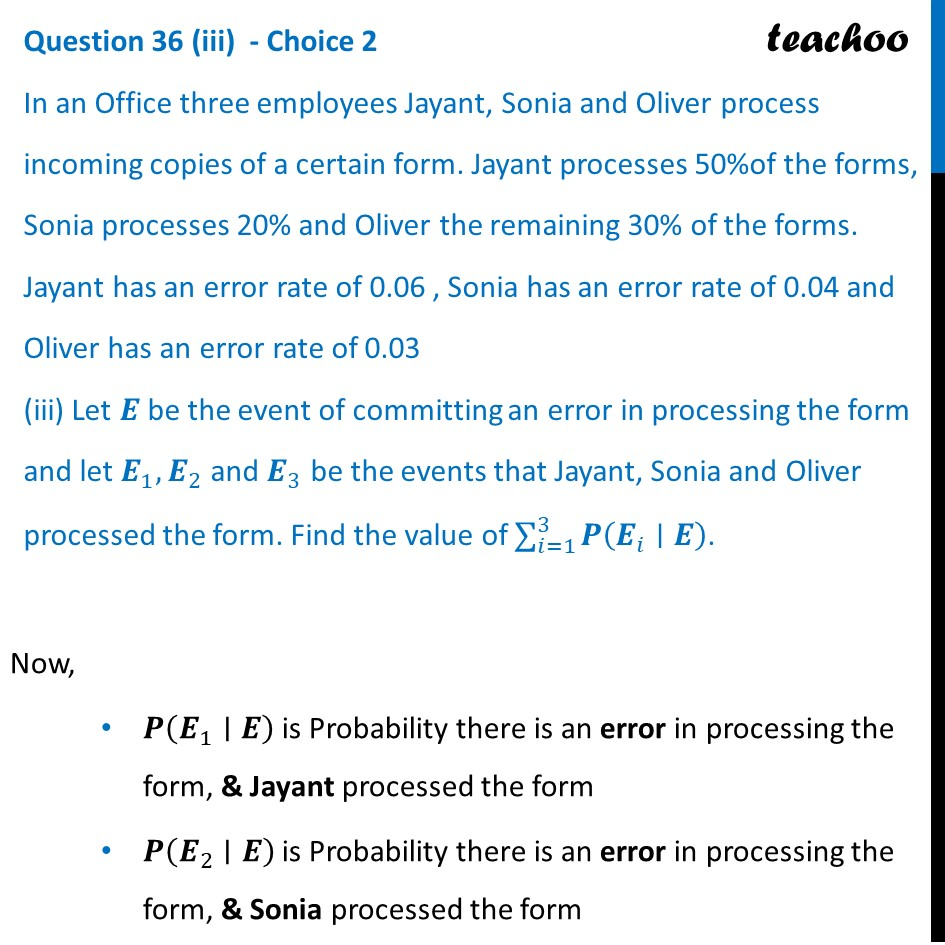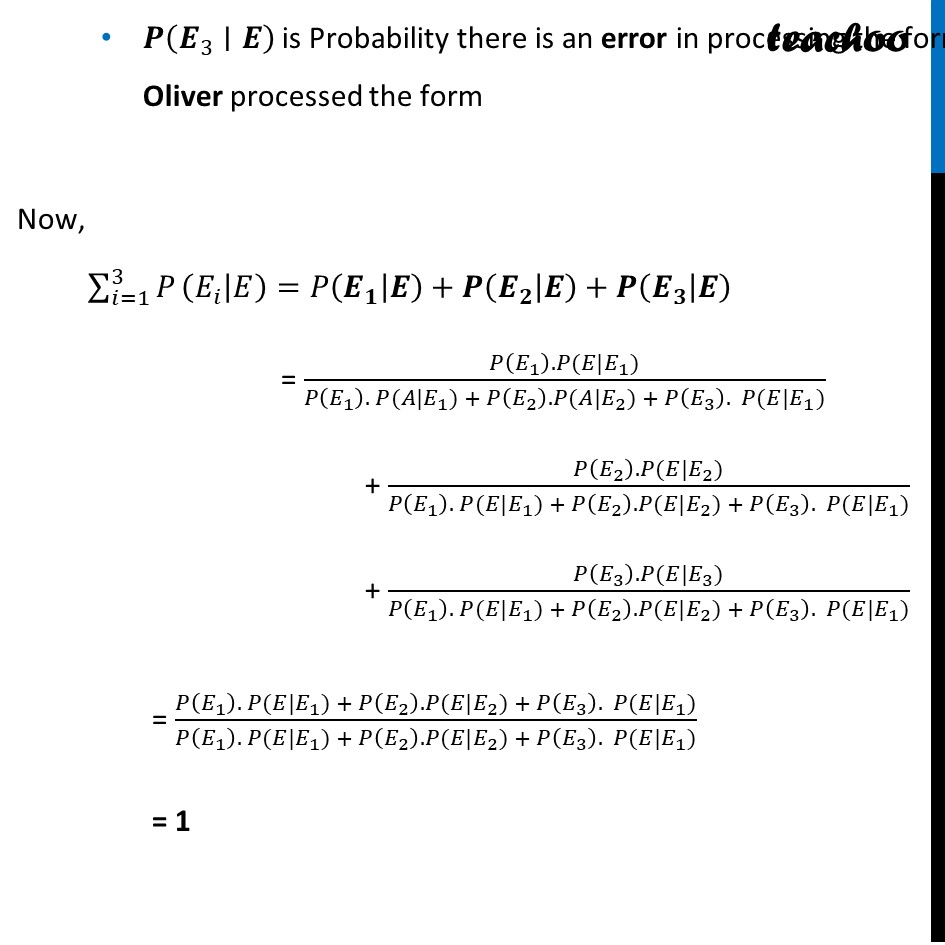CBSE Class 12 Sample Paper for 2024 Boards

Class 12
Solutions of Sample Papers and Past Year Papers - for Class 12 Boards

## Let E  be the event of committing an error in processing the form and let E _ 1, E _ 2 and E _ 3 be the events that Jayant, Sonia and Oliver processed the form. Find the value of ∑ _( i=1 ) ^3  P ( E _ i∣ E) .Learn in your speed, with individual attention - Teachoo Maths 1-on-1 Class

### Transcript

Now, 𝑷(𝑬_1∣𝑬) is Probability there is an error in processing the form, & Jayant processed the form 𝑷(𝑬_2∣𝑬) is Probability there is an error in processing the form, & Sonia processed the form 𝑷(𝑬_3∣𝑬) is Probability there is an error in processing the form, & Oliver processed the form Now, ∑_(𝑖=1)^3▒𝑃 (𝐸_𝑖│𝐸)=𝑃(𝑬_𝟏│𝑬)+𝑷(𝑬_𝟐│𝑬)+𝑷(𝑬_𝟑│𝑬) 𝑷(𝑬_3∣𝑬) is Probability there is an error in processing the form, & Oliver processed the form Now, ∑_(𝑖=1)^3▒𝑃 (𝐸_𝑖│𝐸)=𝑃(𝑬_𝟏│𝑬)+𝑷(𝑬_𝟐│𝑬)+𝑷(𝑬_𝟑│𝑬) = (𝑃(𝐸_1 ). 𝑃(𝐸|𝐸_1))/(𝑃(𝐸_1 ). 𝑃(𝐴|𝐸_1) + 𝑃(𝐸_2 ). 𝑃(𝐴|𝐸_2) + 𝑃(𝐸_3 ). 𝑃(𝐸|𝐸_1)) + (𝑃(𝐸_2 ). 𝑃(𝐸|𝐸_2))/(𝑃(𝐸_1 ). 𝑃(𝐸|𝐸_1) + 𝑃(𝐸_2 ). 𝑃(𝐸|𝐸_2) + 𝑃(𝐸_3 ). 𝑃(𝐸|𝐸_1)) + (𝑃(𝐸_3 ). 𝑃(𝐸|𝐸_3))/(𝑃(𝐸_1 ). 𝑃(𝐸|𝐸_1) + 𝑃(𝐸_2 ). 𝑃(𝐸|𝐸_2) + 𝑃(𝐸_3 ). 𝑃(𝐸|𝐸_1)) = (𝑃(𝐸_1 ). 𝑃(𝐸|𝐸_1) + 𝑃(𝐸_2 ). 𝑃(𝐸|𝐸_2) + 𝑃(𝐸_3 ). 𝑃(𝐸|𝐸_1))/(𝑃(𝐸_1 ). 𝑃(𝐸|𝐸_1) + 𝑃(𝐸_2 ). 𝑃(𝐸|𝐸_2) + 𝑃(𝐸_3 ). 𝑃(𝐸|𝐸_1)) = 1